# RD Sharma Solutions For Class 12 Maths Exercise 19.4 Chapter 19 Indefinite Integrals

RD Sharma Solutions for Class 12 Maths Exercise 19.4 Chapter 19 Indefinite Integrals is provided here. This exercise contains questions pertaining to the evaluation of integrals of the form

$$\begin{array}{l}\frac{P(x)}{(ax+b)^{n}}, n \epsilon N\end{array}$$
where P(x) is a polynomial.

Students wishing to strengthen their concepts can access the RD Sharma Solutions Class 12. To know the right ways to solve questions of this exercise, students can download RD Sharma Solutions for Class 12 Maths Chapter 19 Indefinite Integrals Exercise 19.4, PDF provided below.

## Download the PDF of RD Sharma Solutions For Class 12 Chapter 19 – Indefinite Integrals Exercise 19.4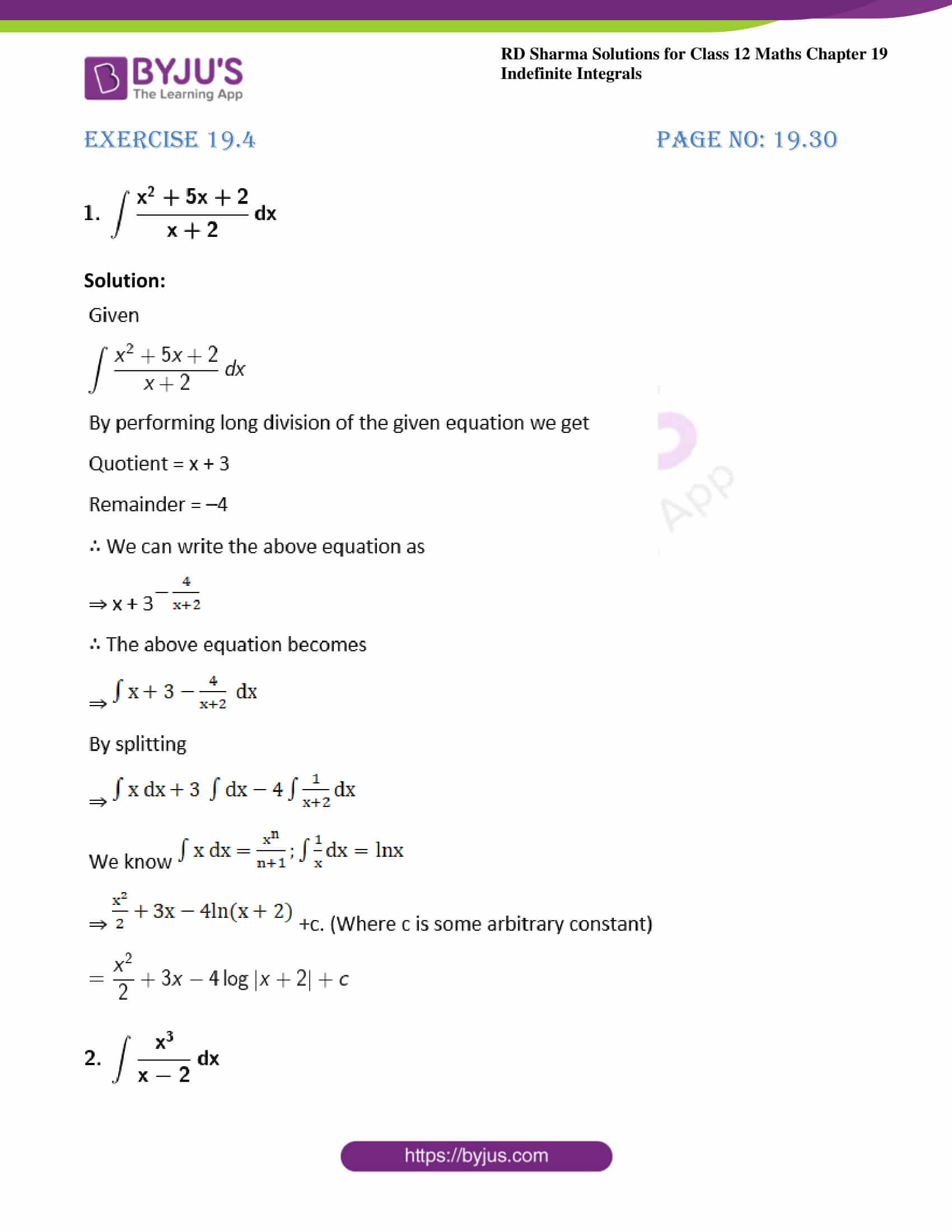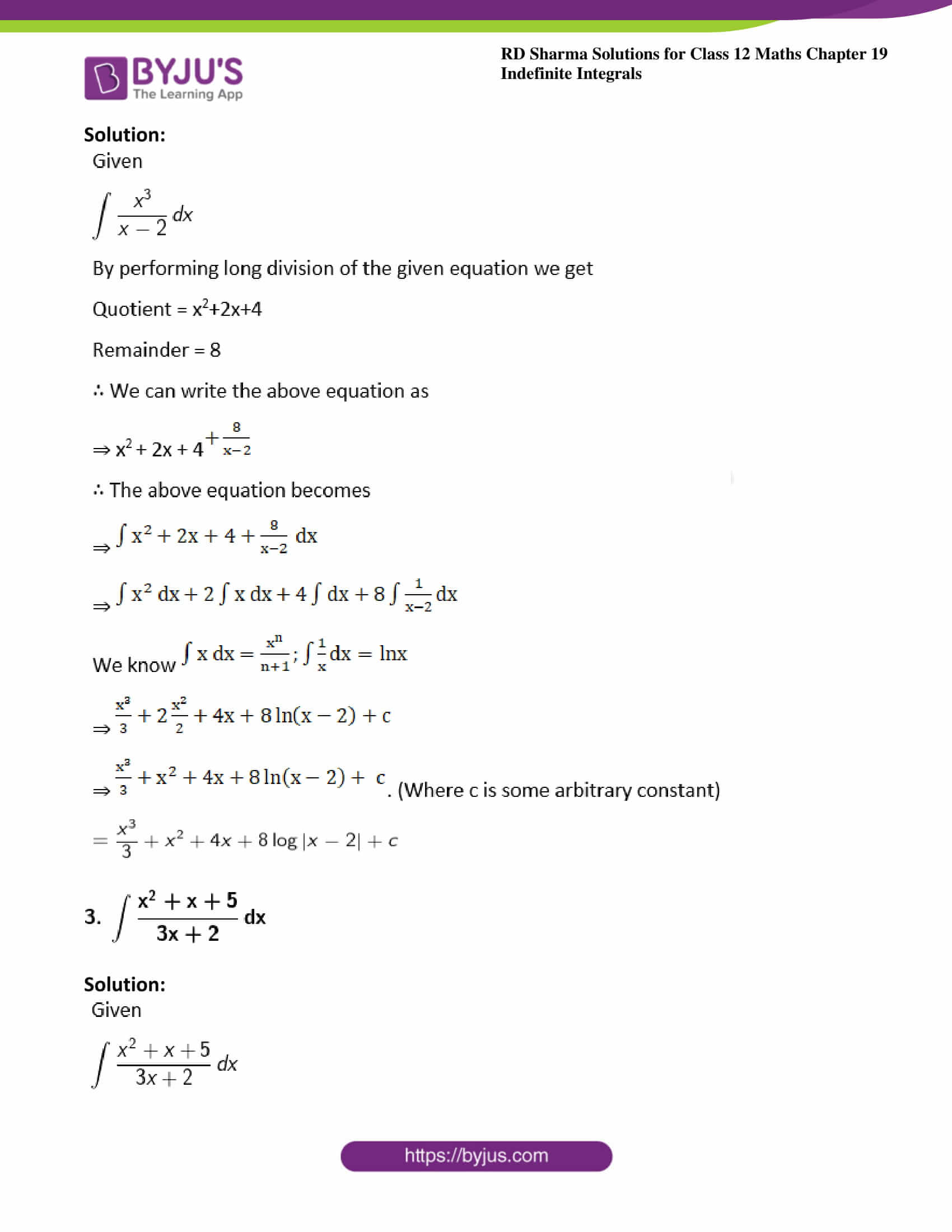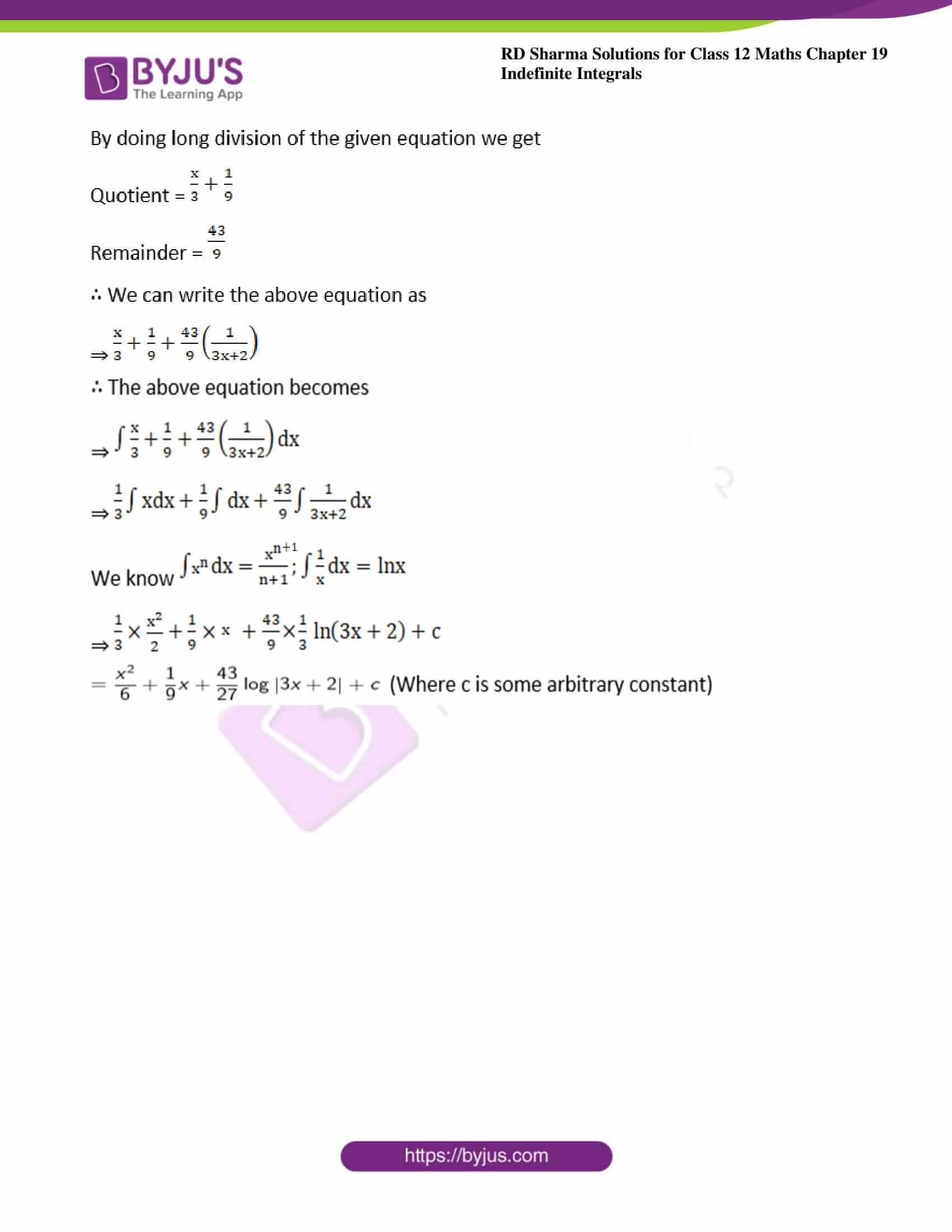### Exercise 19.4 Page No: 19.30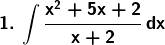Solution: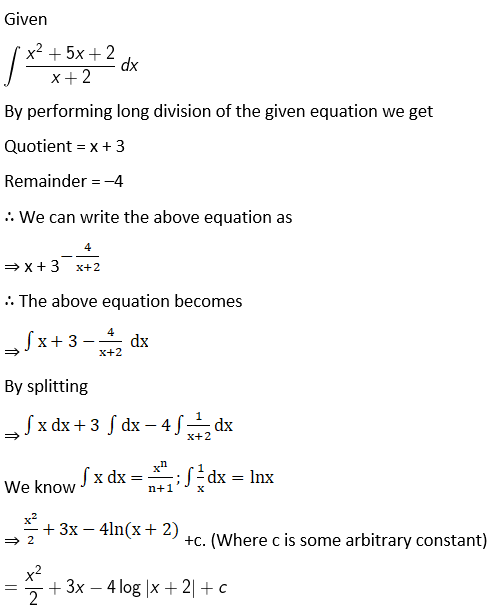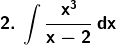Solution: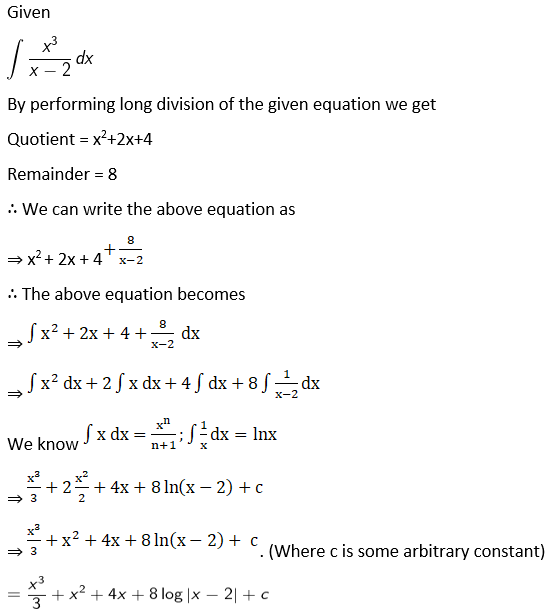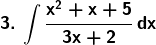Solution: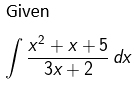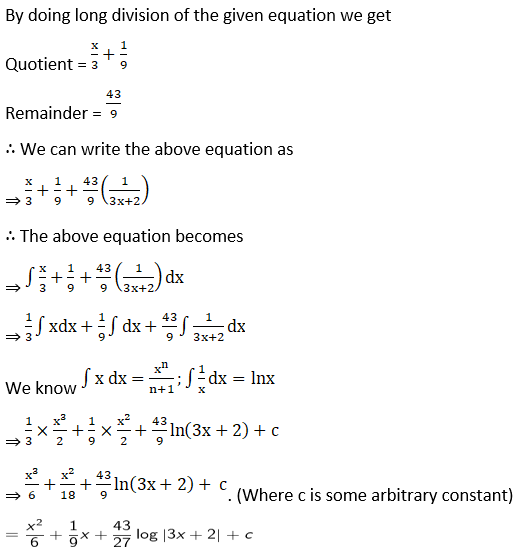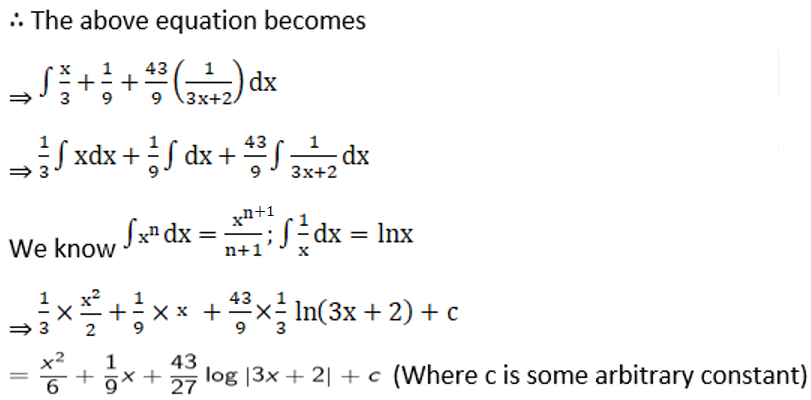### Access other exercises of RD Sharma Solutions For Class 12 Chapter 19 – Indefinite Integrals

Exercise 19.1 Solutions

Exercise 19.2 Solutions

Exercise 19.3 Solutions

Exercise 19.5 Solutions

Exercise 19.6 Solutions

Exercise 19.7 Solutions

Exercise 19.8 Solutions

Exercise 19.9 Solutions

Exercise 19.10 Solutions

Exercise 19.11 Solutions

Exercise 19.12 Solutions

Exercise 19.13 Solutions

Exercise 19.14 Solutions

Exercise 19.15 Solutions

Exercise 19.16 Solutions

Exercise 19.17 Solutions

Exercise 19.18 Solutions

Exercise 19.19 Solutions

Exercise 19.20 Solutions

Exercise 19.21 Solutions

Exercise 19.22 Solutions

Exercise 19.23 Solutions

Exercise 19.24 Solutions

Exercise 19.25 Solutions

Exercise 19.26 Solutions

Exercise 19.27 Solutions

Exercise 19.28 Solutions

Exercise 19.29 Solutions

Exercise 19.30 Solutions

Exercise 19.31 Solutions

Exercise 19.32 Solutions